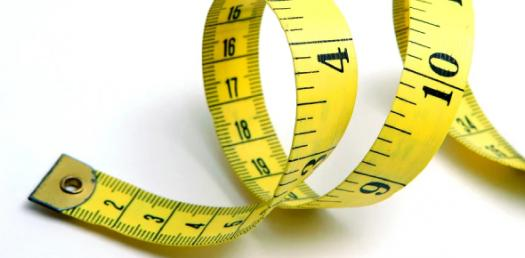# Temperature Measurement Quiz

10 Questions | Total Attempts: 1240SettingsQuestions on the basic operation of fluid thermometers, bimetallic thermometers, thermocouples, and resistance temperature detectors (RTD's).

• 1.
"Which of these forms of energy is the same as heat?"
• A.

A. Thermal energy

• B.

B. Reactive energy

• C.

C. Terminal energy

• D.

D. Resistive energy

• 2.
"Which of these choices best describes what temperature is?"
• A.

A. The number of fast-moving molecules in a substance

• B.

B. The degree, or intensity, of heat in a substance

• C.

C. The ratio of Celsius energy to Fahrenheit energy

• D.

D. The amount of Celsius energy

• 3.
"Which statement best describes the basic operating principle of a fluid thermometer?"
• A.

A. Most fluids contract when they're heated and expand when they're cooled.

• B.

B. Any fluid that's sealed in glass will give off heat.

• C.

C. Most fluids expand when they're heated, and contract when they're cooled.

• D.

D. Most fluids change color when they're heated.

• 4.
"Select all of the actions that will occur when heat is applied to this thermometer.
• A.

A. The bimetallic element expands and rotates.

• B.

B. The rod produces a small voltage.

• C.

C. The rod turns and moves a pointer.

• D.

D. The scale moves.

• 5.
"What happens when heat is applied to the joined ends of the wires of a thermocouple?"
• A.

A. The wires contract.

• B.

B. The wires start to rotate.

• C.

C. The wires generate a small voltage.

• D.

D. The wires separate.

• 6.
"The degree, or intensity, of heat in a substance is known as what?"
• A.

A. Resistance

• B.

B. Temperature

• C.

C. Capacitance

• D.

D. Turbidity

• 7.
"Which  scales are commonly  used  on temperature measuring devices?"
• A.

A. Fahrenheit and Celsius

• B.

B. Fahrenheit and inches of mercury

• C.

C. Celsius and millimeters of mercury

• D.

D. Celsius and PSIA

• 8.
"Select the statement that best describes the principle on which a fluid thermometer operates."
• A.

A. Most fluids contract when they're heated and expand when they're cooled.

• B.

B. Fluids change resistance when temperature changes.

• C.

C. Most fluids expand when they're heated and contract when they're cooled.

• D.

D. Fluids are not affected by changes in temperature.

• 9.
"What is another name that can be used to describe this thermocouple?"
• A.

A. Thermoelectric device

• B.

B. Pressure transmitting device

• C.

C. Metallic diaphragm device

• D.

D. Resistance pressure device

• 10.
"Select all of the actions that occur when the temperature surrounding an RTD increases.
• A.

A. The resistance of the RTD changes.

• B.

B. The circuit detects a change in the current flow.

• C.

C. The wire in the RTD contracts.

• D.

D. The circuit increases voltage to the RTD.

Related TopicsBack to top